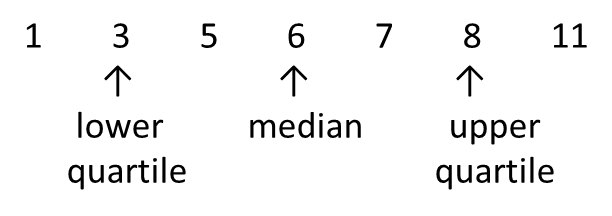# 8.2.3 Interquartile Range

(A) Interquartile Range of Ungrouped DataExample 1:
Find the interquartile range of the following data.
(a) 7, 5, 1, 3, 6, 11, 8
(b) 12, 4, 6, 18, 9, 16, 2, 14

Solution:
(a) Rearrange the data according to ascending order.Interquartile Range
= upper quartile – lower quartile
= 8 – 3
= 5

(b) Rearrange the data according to ascending order.Interquartile Range
= upper quartile – lower quartile
$\frac{14+16}{2}-\frac{4+6}{2}$
= 15 – 5
= 10